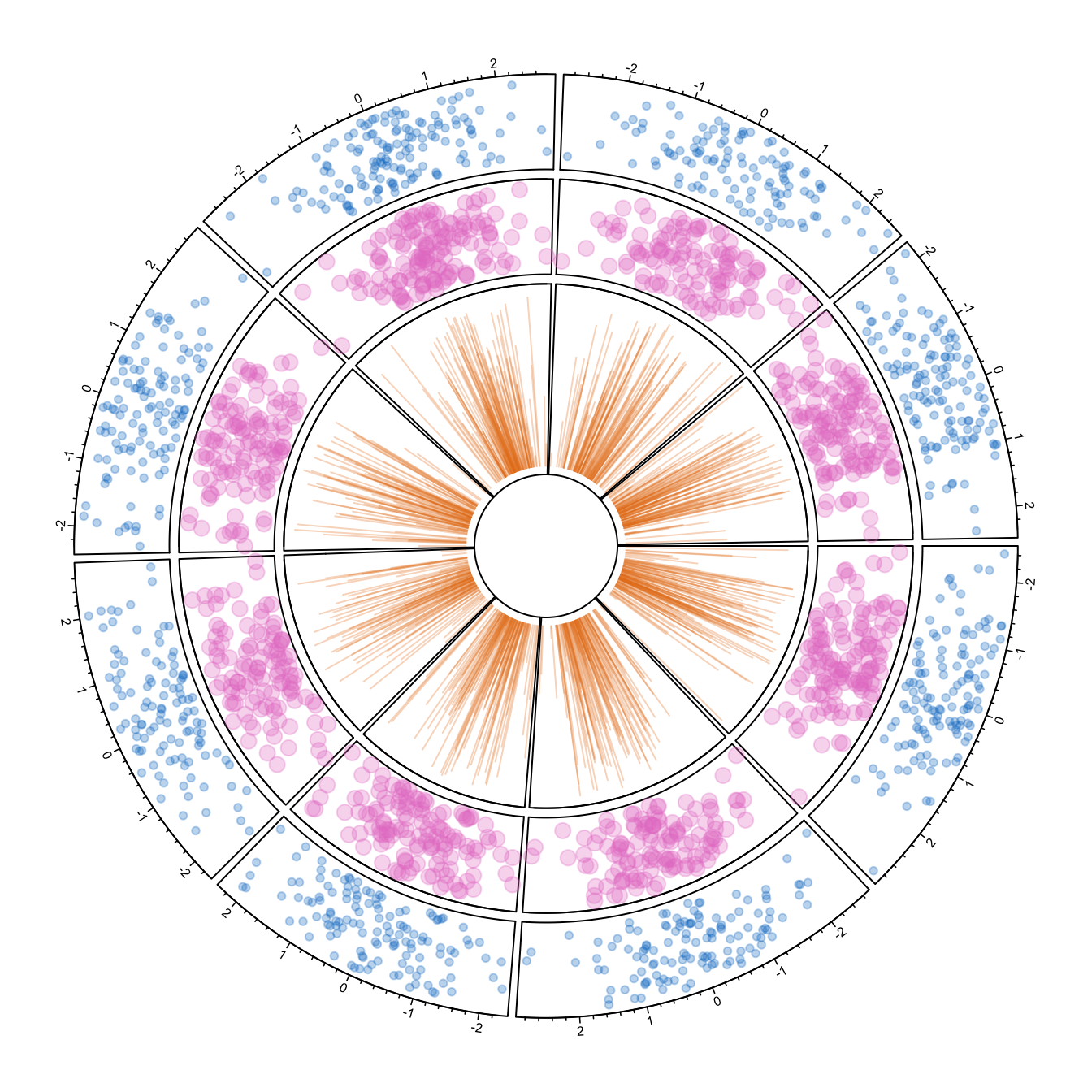# Circular chart with several tracks

The `circlize` package allows to build circular charts with several tracks. This post describes how, providing explanation and reproducible code..

If you read the graph #224, and #225 you know how to make circular plots and customize them.

It is possible to add several tracks on your circular plot: just repeat the step 2 (building regions with `circos.trackPlotRegion`) and 3 (adding dots with `circos.trackPoints` for instance).

Note that you can custom the width of each track using `track.height`.``````#library
library(circlize)
circos.clear()

#Create data
data = data.frame(
factor = sample(letters[1:8], 1000, replace = TRUE),
x = rnorm(1000),
y = runif(1000)
)

#Initialize the plot.
par(mar = c(1, 1, 1, 1) )
circos.initialize(factors = data\$factor, x = data\$x )

# Build the regions of track #1
circos.trackPlotRegion(factors = data\$factor, y=data\$y, panel.fun = function(x, y) {
circos.axis(labels.cex=0.5, labels.font=1, lwd=0.8)
})
# --> Add a scatterplot on it:
circos.trackPoints(data\$factor, data\$x, data\$y, col = rgb(0.1,0.5,0.8,0.3), pch=20)

# Build the regions of track #2:
circlize::circos.trackPlotRegion(factors = data\$factor, y=data\$y, panel.fun = function(x, y) {
circos.axis(labels=FALSE, major.tick=FALSE)
})
# --> Add a scatterplot on it
circos.trackPoints(data\$factor, data\$x, data\$y, col = rgb(0.9,0.5,0.8,0.3), pch=20, cex=2)

# Add track #3 --> don't forget you can custom the height of tracks!
circos.par("track.height" = 0.4)
circos.trackPlotRegion(factors = data\$factor, y=data\$y, panel.fun = function(x, y) {
circos.axis(labels=FALSE, major.tick=FALSE)
})
circos.trackLines(data\$factor, data\$x, data\$y, col = rgb(0.9,0.5,0.1,0.3), pch=20, cex=2, type="h")
# and continue as long as needed!``````

Related chart types

## Contact

This document is a work by Yan Holtz. Any feedback is highly encouraged. You can fill an issue on Github, drop me a message on Twitter, or send an email pasting yan.holtz.data with gmail.com.